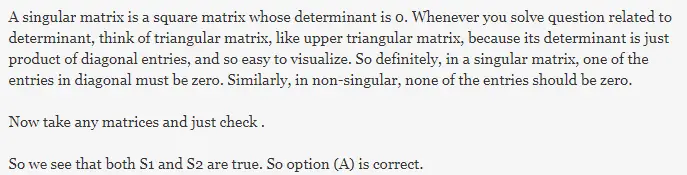# Gate CS-2001 Question Paper With Solutions

Q. 1 Consider the following statements:

```S1: The sum of two singular n × n matrices may be non-singular
S2: The sum of two n × n non-singular matrices may be singular.```

Which of the following statements is correct?

(A) S1 and S2 are both true

(B) S1 is true, S2 is false

(C) S1 is false, S2 is true

(D) S1 and S2 are both false